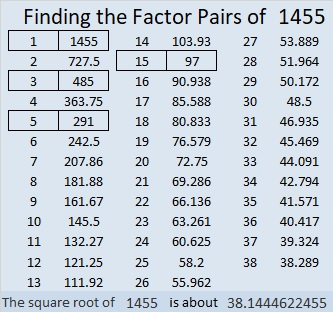# 1455 and Level 1

Contents

### Today’s Puzzle:

All of the clues in today’s puzzle are divisible by the same number. Can you figure out what that number is? If you can, then you can solve this puzzle.### Factors of 1455:

That was puzzle number 1455. In case you would like to know a little bit about that number, here are a few facts:

• 1455 is a composite number.
• Prime factorization: 1455 = 3 × 5 × 97
• 1455 has no exponents greater than 1 in its prime factorization, so √1455 cannot be simplified.
• The exponents in the prime factorization are 1, 1, and 1. Adding one to each exponent and multiplying we get (1 + 1)(1 + 1)(1 + 1) = 2 × 2 × 2 = 8. Therefore 1455 has exactly 8 factors.
• The factors of 1455 are outlined with their factor pair partners in the graphic below.### Facts about the number 1455:

1455 is the difference of two squares in four different ways:
728² – 727² = 1455
244² – 241² = 1455
148² – 143² = 1455
56² – 41² = 1455

1455 is also the hypotenuse of four Pythagorean triples:
132-1449-1455 which is 3 times (44-483-485)
279-1428-1455 which is 3 times (93-476-485)
873-1164-1455 which is (3-4-5) times 291
975-1080-1455 which is 15 times (65-72-97)

This site uses Akismet to reduce spam. Learn how your comment data is processed.